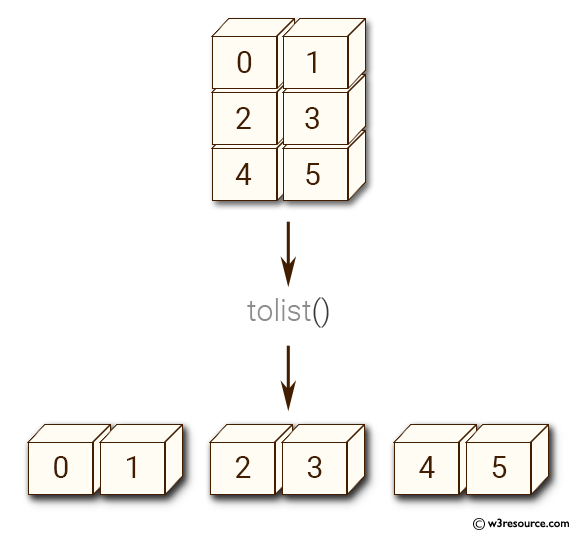﻿ NumPy: Convert a NumPy array into Python list structure - w3resource# NumPy: Convert a NumPy array into Python list structure

## NumPy: Array Object Exercise-80 with Solution

Write a NumPy program to convert a NumPy array into Python list structure.

Pictorial Presentation:Sample Solution:-

Python Code:

``````import numpy as np
x= np.arange(6).reshape(3, 2)
print("Original array elements:")
print(x)
print("Array to list:")
print(x.tolist())
```
```

Sample Output:

```Original array elements:
[[0 1]
[2 3]
[4 5]]
Array to list:
[[0, 1], [2, 3], [4, 5]]

```

Python Code Editor:

Have another way to solve this solution? Contribute your code (and comments) through Disqus.

What is the difficulty level of this exercise?

Test your Python skills with w3resource's quiz

﻿

## Python: Tips of the Day

Set comprehension:

```>>> m = {x ** 2 for x in range(5)}
>>> m
{0, 1, 4, 9, 16}
```